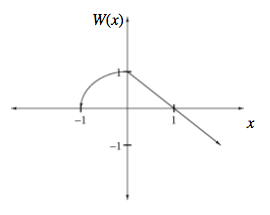Home > A2C > Chapter 8 > Lesson 8.1.2 > Problem8-32

8-32.
1. A function W (x) is sketched below. Homework Help ✎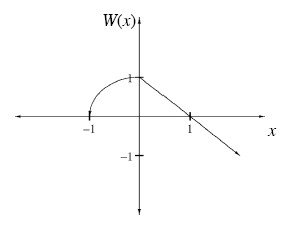1. Make your own copy of the graph, and then sketch the graph of the inverse of W (x).

2. Is the inverse a function? Explain.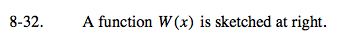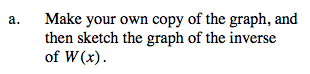Reflect the graph over the line y = x.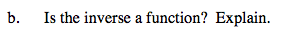For every x-value, is there only one corresponding y-value?